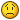# Physics update framerate is fixed or variable?

## Recommended Posts

Hello,

Is the framerate of the physics the same as rendering framerate? I came to this conclusion, because in the GameState class the update callback slows down with rendering, and I can't find a callback for physics update.

I really need a fixed framerate callback and fixed framerate physics here is why:

> I apply a force to a body, the effect of the force does depend on time until next frame (dt), so this time should be fixed, not variable, otherwise I can't calculate the effect of the force (velocity change, dv = F/m * dt )

> I cannot change the velocity instead of applying a force: because I'm using constraints and changing the velocity directly makes the constraints go crazy ( really)

Any idea what can I do?

Ps: I'm using P2 physics.

Edit: the body.thrust(speed) function does something like I want, but the input is a number, I need a similar function with a 2d vector input.

##### Share on other sites

Have you tried this? First argument is your force vector, x and y denote the point where it is applied (0, 0 = center of mass)

`body.applyForce(p2.vec2.fromValues(vectorX, vectorY), x, y);`
##### Share on other sites

Yes I'm using body.applyForce my problem with it is the following:

The applied force is integrated for dt (delta t) time to cause a change in velocity (dv):

dv = F/m * dt

(F: force, m: mass)

But to calculate dv, I need to know dt (the physics update timestep) in advance.

##### Share on other sites

So how does body.thrust do what you want? It's the same as applyForce under the hood...

The docs say for thrust:

"Applies a force to the Body that causes it to 'thrust' forwards, based on its current angle and the given speed. The speed is represented in pixels per second. So a value of 100 would move 100 pixels in 1 second (1000ms)."

This means, after 1 second the sprite's speed would be 100pixels/sec.

This is not exactly what I want, but similar to it:

What I want is to have (initial) v0 + dv speed at the next physics update. As I wrote earlier, I can't set the velocity of the sprite directly because of the constraints. All I need is to know the time until next physics frame.

##### Share on other sites

Well, it should be your fps - ideally 60 so 1/60 seconds.

As far as I know, applyForce works in p2 units per second, which is -0.05 times pixels per second.